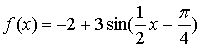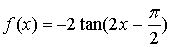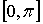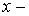King Fahd University of Petroleum and Minerals

Faculty of Science –  Math Per -Year Program

Math 002- term 023

Quiz # 4

Name :                                            ID:                                                   Sec.:

Question1:

Consider the functiona) Find the amplitude

b) Find the period

c) Find the vertical translation

d) Find the phase shift

e) Find the range

f) Graph the function over a complete periodQuestion2:

Consider  the functiona) Find the period

b) Find the equation of the vertical asymptotes over the interval.

c) Find theintercept (s) over the interval.